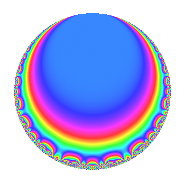Properties

 Label 8048.2.a.lLevel 8048 Weight 2 Character orbit 8048.a Self dual Yes Analytic conductor 64.264 Analytic rank 1 Dimension 2 CM No Inner twists 1

Related objects

Newspace parameters

 Level: $$N$$ = $$8048 = 2^{4} \cdot 503$$ Weight: $$k$$ = $$2$$ Character orbit: $$[\chi]$$ = 8048.a (trivial)

Newform invariants

 Self dual: Yes Analytic conductor: $$64.2636035467$$ Analytic rank: $$1$$ Dimension: $$2$$ Coefficient field: $$\Q(\sqrt{5})$$ Coefficient ring: $$\Z[a_1, a_2, a_3]$$ Coefficient ring index: $$2$$ Fricke sign: $$1$$ Sato-Tate group: $\mathrm{SU}(2)$

$q$-expansion

Coefficients of the $$q$$-expansion are expressed in terms of $$\beta = \sqrt{5}$$. We also show the integral $$q$$-expansion of the trace form.

 $$f(q)$$ $$=$$ $$q$$ $$-\beta q^{3}$$ $$+ ( -1 + \beta ) q^{5}$$ $$+ ( 2 + \beta ) q^{7}$$ $$+ 2 q^{9}$$ $$+O(q^{10})$$ $$q$$ $$-\beta q^{3}$$ $$+ ( -1 + \beta ) q^{5}$$ $$+ ( 2 + \beta ) q^{7}$$ $$+ 2 q^{9}$$ $$+ ( 2 + \beta ) q^{11}$$ $$+ ( -1 - 2 \beta ) q^{13}$$ $$+ ( -5 + \beta ) q^{15}$$ $$+ 2 q^{17}$$ $$+ ( 1 + 3 \beta ) q^{19}$$ $$+ ( -5 - 2 \beta ) q^{21}$$ $$-\beta q^{23}$$ $$+ ( 1 - 2 \beta ) q^{25}$$ $$+ \beta q^{27}$$ $$+ ( -5 + \beta ) q^{29}$$ $$+ ( -1 - 3 \beta ) q^{31}$$ $$+ ( -5 - 2 \beta ) q^{33}$$ $$+ ( 3 + \beta ) q^{35}$$ $$-2 \beta q^{37}$$ $$+ ( 10 + \beta ) q^{39}$$ $$+ ( -3 - \beta ) q^{41}$$ $$+ ( -8 - \beta ) q^{43}$$ $$+ ( -2 + 2 \beta ) q^{45}$$ $$+ ( -2 - \beta ) q^{47}$$ $$+ ( 2 + 4 \beta ) q^{49}$$ $$-2 \beta q^{51}$$ $$+ ( 4 - 2 \beta ) q^{53}$$ $$+ ( 3 + \beta ) q^{55}$$ $$+ ( -15 - \beta ) q^{57}$$ $$-4 \beta q^{59}$$ $$+ ( 3 + 2 \beta ) q^{61}$$ $$+ ( 4 + 2 \beta ) q^{63}$$ $$+ ( -9 + \beta ) q^{65}$$ $$+ ( 2 - 3 \beta ) q^{67}$$ $$+ 5 q^{69}$$ $$+ ( -3 + \beta ) q^{71}$$ $$+ ( -12 + 2 \beta ) q^{73}$$ $$+ ( 10 - \beta ) q^{75}$$ $$+ ( 9 + 4 \beta ) q^{77}$$ $$+ ( -4 - 4 \beta ) q^{79}$$ $$-11 q^{81}$$ $$+ ( -10 - 3 \beta ) q^{83}$$ $$+ ( -2 + 2 \beta ) q^{85}$$ $$+ ( -5 + 5 \beta ) q^{87}$$ $$+ ( -10 - 2 \beta ) q^{89}$$ $$+ ( -12 - 5 \beta ) q^{91}$$ $$+ ( 15 + \beta ) q^{93}$$ $$+ ( 14 - 2 \beta ) q^{95}$$ $$-10 q^{97}$$ $$+ ( 4 + 2 \beta ) q^{99}$$ $$+O(q^{100})$$ $$\operatorname{Tr}(f)(q)$$ $$=$$ $$2q$$ $$\mathstrut -\mathstrut 2q^{5}$$ $$\mathstrut +\mathstrut 4q^{7}$$ $$\mathstrut +\mathstrut 4q^{9}$$ $$\mathstrut +\mathstrut O(q^{10})$$ $$2q$$ $$\mathstrut -\mathstrut 2q^{5}$$ $$\mathstrut +\mathstrut 4q^{7}$$ $$\mathstrut +\mathstrut 4q^{9}$$ $$\mathstrut +\mathstrut 4q^{11}$$ $$\mathstrut -\mathstrut 2q^{13}$$ $$\mathstrut -\mathstrut 10q^{15}$$ $$\mathstrut +\mathstrut 4q^{17}$$ $$\mathstrut +\mathstrut 2q^{19}$$ $$\mathstrut -\mathstrut 10q^{21}$$ $$\mathstrut +\mathstrut 2q^{25}$$ $$\mathstrut -\mathstrut 10q^{29}$$ $$\mathstrut -\mathstrut 2q^{31}$$ $$\mathstrut -\mathstrut 10q^{33}$$ $$\mathstrut +\mathstrut 6q^{35}$$ $$\mathstrut +\mathstrut 20q^{39}$$ $$\mathstrut -\mathstrut 6q^{41}$$ $$\mathstrut -\mathstrut 16q^{43}$$ $$\mathstrut -\mathstrut 4q^{45}$$ $$\mathstrut -\mathstrut 4q^{47}$$ $$\mathstrut +\mathstrut 4q^{49}$$ $$\mathstrut +\mathstrut 8q^{53}$$ $$\mathstrut +\mathstrut 6q^{55}$$ $$\mathstrut -\mathstrut 30q^{57}$$ $$\mathstrut +\mathstrut 6q^{61}$$ $$\mathstrut +\mathstrut 8q^{63}$$ $$\mathstrut -\mathstrut 18q^{65}$$ $$\mathstrut +\mathstrut 4q^{67}$$ $$\mathstrut +\mathstrut 10q^{69}$$ $$\mathstrut -\mathstrut 6q^{71}$$ $$\mathstrut -\mathstrut 24q^{73}$$ $$\mathstrut +\mathstrut 20q^{75}$$ $$\mathstrut +\mathstrut 18q^{77}$$ $$\mathstrut -\mathstrut 8q^{79}$$ $$\mathstrut -\mathstrut 22q^{81}$$ $$\mathstrut -\mathstrut 20q^{83}$$ $$\mathstrut -\mathstrut 4q^{85}$$ $$\mathstrut -\mathstrut 10q^{87}$$ $$\mathstrut -\mathstrut 20q^{89}$$ $$\mathstrut -\mathstrut 24q^{91}$$ $$\mathstrut +\mathstrut 30q^{93}$$ $$\mathstrut +\mathstrut 28q^{95}$$ $$\mathstrut -\mathstrut 20q^{97}$$ $$\mathstrut +\mathstrut 8q^{99}$$ $$\mathstrut +\mathstrut O(q^{100})$$

Embeddings

For each embedding $$\iota_m$$ of the coefficient field, the values $$\iota_m(a_n)$$ are shown below.

For more information on an embedded modular form you can click on its label.

Label $$\iota_m(\nu)$$ $$a_{2}$$ $$a_{3}$$ $$a_{4}$$ $$a_{5}$$ $$a_{6}$$ $$a_{7}$$ $$a_{8}$$ $$a_{9}$$ $$a_{10}$$
1.1
 1.61803 −0.618034
0 −2.23607 0 1.23607 0 4.23607 0 2.00000 0
1.2 0 2.23607 0 −3.23607 0 −0.236068 0 2.00000 0
 $$n$$: e.g. 2-40 or 990-1000 Significant digits: Format: Complex embeddings Normalized embeddings Satake parameters Satake angles

Inner twists

This newform does not admit any (nontrivial) inner twists.

Atkin-Lehner signs

$$p$$ Sign
$$2$$ $$-1$$
$$503$$ $$-1$$

Hecke kernels

This newform can be constructed as the intersection of the kernels of the following linear operators acting on $$S_{2}^{\mathrm{new}}(\Gamma_0(8048))$$:

 $$T_{3}^{2}$$ $$\mathstrut -\mathstrut 5$$ $$T_{5}^{2}$$ $$\mathstrut +\mathstrut 2 T_{5}$$ $$\mathstrut -\mathstrut 4$$ $$T_{7}^{2}$$ $$\mathstrut -\mathstrut 4 T_{7}$$ $$\mathstrut -\mathstrut 1$$ $$T_{13}^{2}$$ $$\mathstrut +\mathstrut 2 T_{13}$$ $$\mathstrut -\mathstrut 19$$